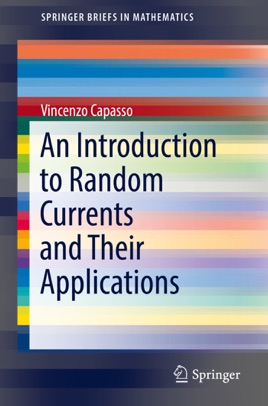• USD 54.99

## Descripción de editorial

This book introduces random currents by presenting underlying mathematical methods necessary for applications. The theory of currents is an advanced topic in geometric measure theory that extends distribution to linear functionals within the space of differential forms of any order. Methods to extend random distributions to random currents are introduced and analyzed in this book.  Beginning with an overview of mathematical aspects of the theory of currents, this book moves on to examine applications in medicine, material science, and image analysis.  Applied researchers will find the practical modern mathematical methods along with the detailed appendix useful to stimulate new applications and research.